# Subtraction of five digit numbers | Maths Grade 4

Learn about Subtraction of five digit numbers, 5 digit subtraction without borrowing, 5 digit subtraction with borrowing

## Subtraction of five digit numbers without borrowing

Question 1

Subtract the following:Explanation

Subtracting the digits at ones place
5 – 3 = 2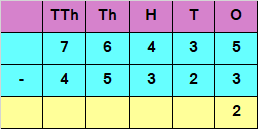Subtracting the digits at tens place
3 – 2 = 1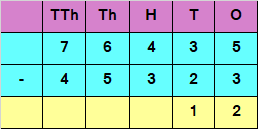Subtracting the digits at hundreds place
4 – 3 = 1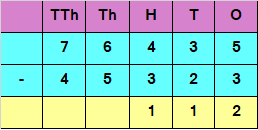Subtracting the digits at thousands place
6 – 5 = 1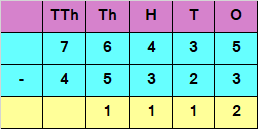Subtracting the digits at ten thousands place
7 – 4 = 3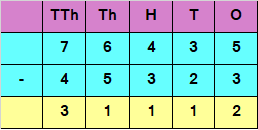Hence, the difference is 31112

Question 2

Subtract the following: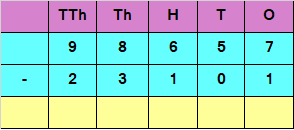Explanation

Subtracting the digits at ones place
7 – 1 = 6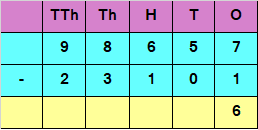Subtracting the digits at tens place
5 – 0 = 5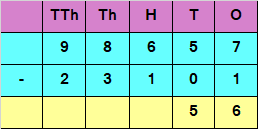Subtracting the digits at hundreds place
6 – 1 = 5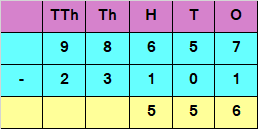Subtracting the digits at thousands place
8 – 3 = 5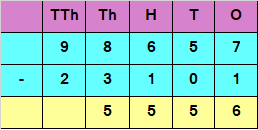Subtracting the digits at ten thousands place
9 – 2 = 7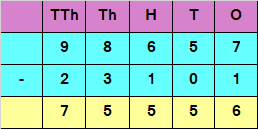Hence, the difference is 75556

## Subtraction of five digit numbers with borrowing

Question 1

Subtract the following: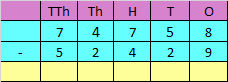Explanation

If we want to Subtract the digits at ones place, we have
8 – 9
But 9 is greater than 8
We would need to borrow 1 from 5 in tens place
8 in ones place would now become 18 and 5 in tens place would be reduced to 4
So, 18 – 9 = 9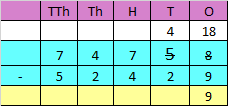Subtracting the digits at tens place
4 – 2 = 2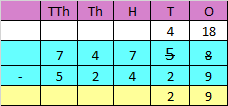Subtracting the digits at hundreds place
7 – 4 = 3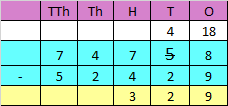Subtracting the digits at thousands place
4 – 2 = 2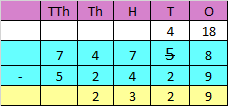Subtracting the digits at ten thousands place
7 – 5 = 2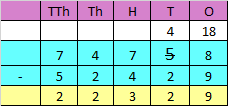Hence, the difference is 22329

Question 2

Subtract the following: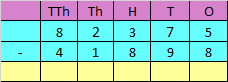If we want to Subtract the digits at ones place, we have
5 – 8
But 8 is greater than 5
We would need to borrow 1 from 7 in tens place
5 in ones place would now become 15 and 7 in tens place would be reduced to 6
So, 15 – 8 = 7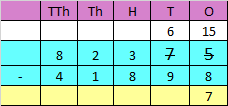If we want to Subtract the digits at tens place, we have
6 – 9
But 9 is greater than 6
We would need to borrow 1 from 3 in hundred place
6 in tens place would now become 16 and 3 in hundred place would be reduced to 2
So, 16 – 9 = 7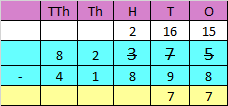If we want to Subtract the digits at hundred place, we have
2 – 8
But 8 is greater than 2
We would need to borrow 1 from 2 in thousand place
2 in hundred place would now become 12 and 2 in thousand place would be reduced to 1
So, 12 – 8 = 4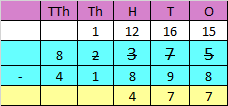Subtracting the digits at thousands place
1 – 1 = 0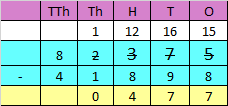Subtracting the digits at ten thousands place
8 – 4 = 4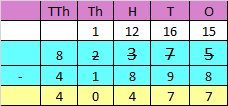Hence, the difference is 40477